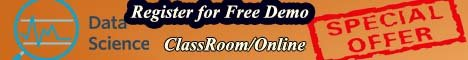# Best institute for DATA SCIENCE training IN  HYDERABAD

## Introduction to Data Science

• Why it is important and who are eligible### Use Cases/Business Application (Retail, CPG, Banking, Telecom etc.)

• Different scenario where DS can be applied to solve business problems

### Basics of Statistics –

• Descriptive Statistics for
• Mean, Median, Mode, Quartile, Percentile, Inter-Quartile Range
• Standard Deviation, Variance
• Descriptive Statistics for two variables
• Z-Score
• Co-variance, Co-relation
• Chi-squared Analysis
• Hypothesis Testing

### Probability concepts –

• Basic Probability, Conditional Probability
• Properties of Random Variables
• Expectations, Variance
• Entropy and cross-entropy
• Estimating probability of Random variable
• Understanding standard random processes### Data Distributions

• Normal Distribution
• Binomial Distribution
• Multinomial Distribution
• Bernoulli Distribution
• Probability, Prior probability, Posterior probability
• Naive Bayes Algorithm

### Basic Mathematics for Data Science

• Limits,
• Derivatives, Partial Derivatives
• Gradients, Significance of Gradients

### Mastering Python/R Language

• How to install python (Anaconda), sciKit Learn
• How to work with Jupyter Notebook and Spyder IDE
• Strings, Lists, Tuples, and Sets
• Dictionaries, Control Flows, Functions
• Formal/Positional/Keyword arguments
• Predefined functions (range, len, enumerates etc…)
• Data Frames
• Packages required for data Science in R/Python### Introduction to NumPy

• One-dimensional Array, Two-dimensional Array
• Pre-defined functions (arrange, reshape, zeros, ones, empty)
• Basic Matrix operations
• Scalar addition, subtraction, multiplication, division
• Matrix addition, subtraction, multiplication, division and transpose
• Slicing, Indexing, Looping
• Shape Manipulation, Stacking

### Introduction to Pandas

• Series, DataFrame, GroupBy
• Crosstab, apply and map

### Data Preparation Techniques

• Applications of PCA: Dimensionality Reduction
• Feature Engineering (FE)
• Combine Features
• Split Features### Data visualization

• Bar Chart
• Histogram
• Box whisker plot
• Line plot
• Scatter Plot and Heat Maps

Machine Learning Algorithm – Data Preparation and Execution

• Linear Regression
• Logistic Regression
• Optimization (Gradient Descent etc.)
• Decision Tree
• Random Forest
• Boosting and AdaBoost
• Clustering Algorithms (KNN and K-Means)
• Support Vector Machines
• Nave Bayes Algorithm
• Neural Networks
• Text Mining (NLTK)
• Introduction to Deep learning

Note: All these Algorithms will be explained using one case study and executed in python.